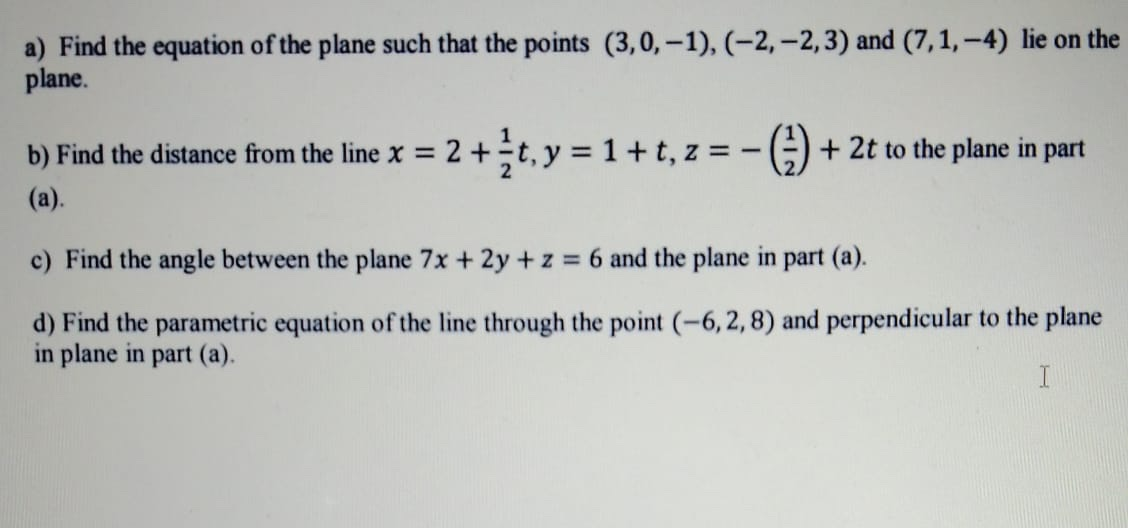2022-01-04please solve this question as soon as possiblealenahelenash

Expert

a) Let the equation be of form,

$a\left(x-3\right)+b\left(y-0\right)+c\left(z-\left(-1\right)\right)=0$

Since it through $\left(-2,-2,3\right)$ and $\left(7,1,-4\right)$

$a\left(-2-3\right)+b\left(-2\right)+c\left(3+1\right)=0$

$⇒5a+2b-3c=0$ --- (1)

$a\left(7-3\right)+b\left(1-0\right)+c\left(-4+1\right)=0$

$4a+b-3c=0$

$5a+2b-3c=0$

$\frac{a}{-3+6}=\frac{b}{-15+12}=\frac{c}{8-5}$

$\frac{a}{3}=\frac{b}{-3}=\frac{c}{3}$

$\because 3\left(x-3\right)-3\left(y\right)+3\left(2+1\right)=0$

$⇒$ $x-y+z=2$ Required plane

b) Since the given line is intersetting with the plane for $t=1$, hence distance $=0$

c) Angle,

$\theta ={\mathrm{cos}}^{-1}\left(\frac{<7,2,1>\cdot <1,-1,1>}{\sqrt{{7}^{2}+{2}^{2}+{1}^{2}}\cdot \sqrt{{1}^{2}+{1}^{2}+{1}^{2}}}\right)$

$={\mathrm{cos}}^{-1}\left(\frac{6}{\sqrt{54}\cdot \sqrt{3}}\right)$

$={\mathrm{cos}}^{-1}\left(\frac{6}{9\sqrt{2}}\right)$

$={\mathrm{cos}}^{-1}\left(\frac{\sqrt{2}}{3}\right)$

d) Since normal of plane will be direction ratio for line,

$x=-6+t,y=2-t,z=8+t$

Do you have a similar question?